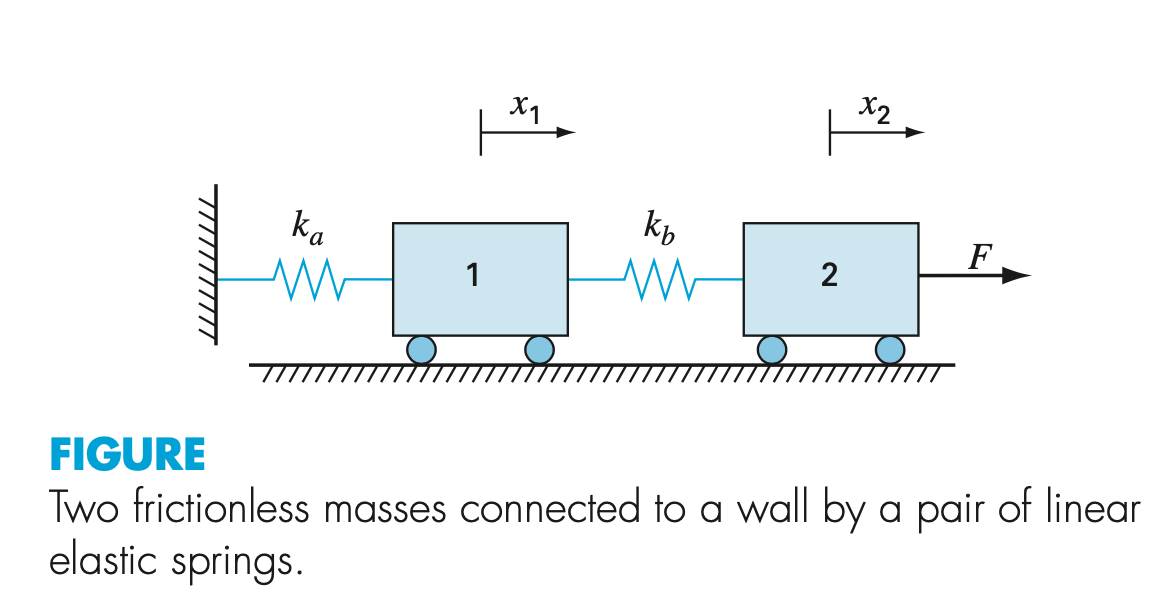# Question Case. Develop a potential energy function for the system illustrated in the figure. Develop the contour and surface graphs using matlab and minimize the potential energy function to determine the equilibrium displacements x1 and x2 given the forcing function F = 100 N, and parameters ka = 20 and kb = 5 N/m. X1 X2 ka k, 77777777777 F 1 M 2 Tur FIGURE Two frictionless masses connected to a wall by a pair of linear elastic springs.6LDBNS The Asker · Computer Science

Case.
Develop a potential energy function for the system illustrated in the figure.Develop the contour and surface graphs using matlab and minimize the potential energy function to determine the equilibrium displacements x1 and x2 given the forcing function F = 100 N, and parameters ka = 20 and kb = 5 N/m.

Transcribed Image Text: X1 X2 ka k, 77777777777 F 1 M 2 Tur FIGURE Two frictionless masses connected to a wall by a pair of linear elastic springs.
More
Transcribed Image Text: X1 X2 ka k, 77777777777 F 1 M 2 Tur FIGURE Two frictionless masses connected to a wall by a pair of linear elastic springs.